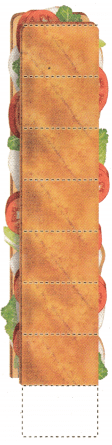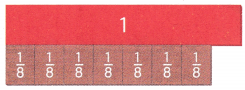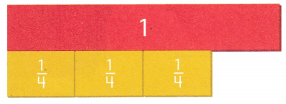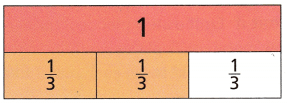# Texas Go Math Grade 4 Lesson 3.5 Answer Key Write Fractions as Sums

Refer to our Texas Go Math Grade 4 Answer Key Pdf to score good marks in the exams. Test yourself by practicing the problems from Texas Go Math Grade 4 Lesson 3.5 Answer Key Write Fractions as Sums.

## Texas Go Math Grade 4 Lesson 3.5 Answer Key Write Fractions as Sums

Unlock the ProblemEmilio cut a sandwich into 8 equal pieces and ate 1 piece. He has $$\frac{7}{8}$$ of the sandwich left. Emilio put each remaining piece on a snack plate. How many snack plates did he use? What part of the sandwich did he put on each plate?

Each piece of the sandwich is $$\frac{1}{8}$$ the whole. $$\frac{1}{8}$$ is called a unit fraction because it tells the part of the whole that 1 piece represents. A unit traction always has a numerator of 1.

Example 1 Use fraction strips. Write $$\frac{7}{8}$$ as a sum of unit fractions.$$\frac{7}{8}$$ = _________ + _________ + _________ + _________ + _________ + _________ + _________

The number of addends represents the number of plates used.
The unit fractions represent the part of the sandwich on each plate.

So, Emilio used _________ plates. He put _________ of a sandwich on each plate.

What if Emilio ate 3 pieces 01 the sandwich instead of 1 piece? How many snack plates would he need? What part of the sandwich would be on each plate? Explain.

Example 2 Write a fraction as a sum. Kevin and Olivia are going to share a whole pizza. The pizza is cut into 6 equal slices. They will put the slices on two separate dishes. What part of the whole pizza could be on each dish?

Shade the models to show how Kevin and Olivia could share the pizza. Write an equation.
Think: $$\frac{6}{6}$$ = 1 whole pizza.Math Talk

Mathematical Processes
Explain how the numerator in $$\frac{5}{6}$$ is related to the number of addends in the sum of its unit fraction.

Share and Show

Question 1.
Write $$\frac{3}{4}$$ as a sum of unit fractions.$$\frac{3}{4}$$ = __________ + __________+ __________

Write the fraction as a sum of unit fractions.

Question 2.$$\frac{5}{6}$$ = __________

Question 3.$$\frac{2}{3}$$ = __________

Question 4.
H.O.T. How many different ways can you write a fraction that has a numerator of 2 as a sum of fractions? Explain.

Problem Solving

Question 5.
H.O.T. Representations Holly’s garden is divided into 5 equal sections. She will fence the garden into 3 areas by grouping some equal sections together. What part of the garden could each fenced area be?

a. What information do you need to use?

d. Show how you can solve the problem.

e. Complete the sentence.
The garden can be fenced into ________, ________, and ________ parts or ________, ________, and ________ parts.

Question 8.
Paula mixed strawberry yogurt and milk to make a smoothie. The smoothie fills $$\frac{2}{3}$$ cup. Which is $$\frac{2}{3}$$ written as the sum of unit fractions?
(A) $$\frac{2}{3}+\frac{2}{3}$$
(B) $$\frac{1}{2}+\frac{1}{2}$$
(C) $$\frac{1}{3}+\frac{2}{3}$$
(D) $$\frac{1}{3}+\frac{1}{3}$$

Question 9.
Use Tools Larry’s dog, Rex, ate $$\frac{3}{4}$$ of a can of dog food. What is another was of writing $$\frac{3}{4}$$? Use fraction strips to answer the question.
(A) $$\frac{1}{4}+\frac{1}{4}$$
(B) $$\frac{1}{4}+\frac{1}{4}+\frac{1}{4}$$
(C) $$\frac{1}{4}+\frac{1}{4}+\frac{1}{4}+\frac{1}{4}$$
(D) $$\frac{3}{4}+\frac{3}{4}+\frac{3}{4}$$

Question 10.
Ben and his friends ate $$\frac{6}{8}$$ of a whole pizza. Written as a sum of unit fractions, which shows the amount of pizza Ben and his friends ate?
(A) $$\frac{1}{8}+\frac{1}{8}+\frac{1}{8}+\frac{1}{8}+\frac{1}{8}+\frac{1}{8}$$
(B) $$\frac{1}{6}+\frac{1}{6}+\frac{1}{6}+\frac{1}{6}+\frac{1}{6}+\frac{1}{6}$$
(C) $$\frac{1}{8}+\frac{1}{8}+\frac{1}{8}+\frac{1}{8}+\frac{1}{8}+\frac{1}{8}+\frac{1}{8}+\frac{1}{8}$$
(D) $$\frac{1}{6}+\frac{1}{6}+\frac{1}{6}+\frac{1}{6}+\frac{1}{6}+\frac{1}{6}+\frac{1}{6}+\frac{1}{6}$$

TEXAS Test Prep

Question 11.
Which is equivalent to $$\frac{9}{12}$$?
(A) $$\frac{5}{12}+\frac{3}{12}$$
(B) $$\frac{3}{12}+\frac{2}{12}+\frac{1}{12}+\frac{1}{12}$$
(C) $$\frac{5}{12}+\frac{2}{12}+\frac{2}{12}$$
(D) $$\frac{4}{12}+\frac{4}{12}+\frac{1}{12}+\frac{1}{12}$$

### Texas Go Math Grade 4 Lesson 3.5 Homework and Practice Answer Key

Question 1.$$\frac{2}{5}$$ = ___________

Question 2.$$\frac{6}{7}$$ = ___________

Question 3.
What is $$\frac{4}{12}$$ written as a sum of unit fractions?

Question 4.
What is $$\frac{6}{8}$$ written as a sum of unit fractions?

Question 5.
What is $$\frac{8}{10}$$ written as a sum of unit fractions?

Question 6.
What is $$\frac{7}{9}$$ written as a sum of unit fractions?

Problem Solving

Question 7.
Hank cut a cake into 12 equal pieces and ate 2 pieces. He has $$\frac{10}{12}$$ of the cake left to serve on plates. What part of the cake did he put on each plate?

Question 8.
How many plates did hank use to serve the cake?

Lesson check

Question 9.
What is $$\frac{5}{6}$$ written as a sum of unit fractions
(A) $$\frac{3}{6}+\frac{1}{6}+\frac{1}{6}$$
(B) $$\frac{1}{6}+\frac{1}{6}+\frac{1}{6}+\frac{2}{6}$$
(C) $$\frac{6}{6}+\frac{1}{6}$$
(D) $$\frac{1}{6}+\frac{1}{6}+\frac{1}{6}+\frac{1}{6}+\frac{1}{6}$$

Question 10.
Which is equivalent to $$\frac{8}{15}$$
(A) $$\frac{5}{15}+\frac{4}{15}$$
(B) $$\frac{4}{15}+\frac{2}{15}+\frac{2}{15}$$
(C) $$\frac{3}{15}+\frac{3}{15}+\frac{4}{15}$$
(D) $$\frac{2}{15}+\frac{2}{15}+\frac{2}{15}+\frac{1}{15}$$

Question 11.
Kay lives $$\frac{5}{8}$$ mile from her friend. Which is $$\frac{5}{8}$$ written as the sum of unit fractions?
(A) $$\frac{1}{8}+\frac{5}{8}$$
(B) $$\frac{1}{8}+\frac{1}{8}+\frac{1}{8}+\frac{1}{8}+\frac{1}{8}$$
(C) $$\frac{5}{8}+\frac{5}{8}$$
(D) $$\frac{1}{8}+\frac{1}{8}+\frac{1}{8}+\frac{1}{8}$$

Question 12.
Which is equivalent to $$\frac{7}{12}$$?
(A) $$\frac{1}{12}+\frac{1}{12}+\frac{1}{12}+\frac{1}{12}+\frac{1}{12}$$
(B) $$\frac{7}{12}+\frac{1}{12}$$
(C) $$\frac{5}{12}+\frac{3}{12}$$
(D) 

Question 13.
Multi-Step Leena walked $$\frac{2}{3}$$ of a mile. What is $$\frac{2}{3}$$ written as a sum of unit fractions with a denominator of 9?
(A) $$\frac{5}{9}+\frac{4}{9}$$
(B) $$\frac{1}{9}+\frac{1}{9}+\frac{1}{9}+\frac{1}{9}+\frac{1}{9}+\frac{1}{9}$$
(C) $$\frac{2}{9}+\frac{2}{9}+\frac{2}{9}$$
(D) $$\frac{1}{9}+\frac{1}{9}+\frac{1}{9}$$
Multi-Step William waters his plants on different days. He watered $$\frac{1}{5}$$ of the plants on Monday and $$\frac{1}{5}$$ of the plants on Tuesday. What fraction shows the part of the plants that William still needs to water this week?
(A) $$\frac{3}{5}$$
(B) $$\frac{2}{5}$$
(C) $$\frac{1}{5}$$
(D) $$\frac{4}{5}$$